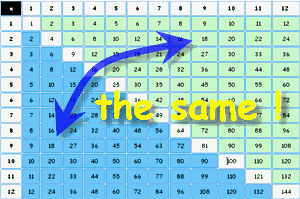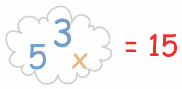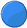## How to Learn

Your life will be a lot easier when you can simply remember the multiplication tables.

So, the two main ways for you to learn the multiplication table are:

• Exercising using the Math Trainer

## Tip 1: Order Does Not Matter

 When we multiply two numbers, it does not matter which is first or second, the answer is always the same.

Example: 3×5=15, and 5×3=15

Another Example: 2×9=18, and 9×2=18In fact half of the table is a mirror image of the other!

So, don’t memorize both “3×5” and “5×3”, just memorize that “a 3 and a 5 make 15” when multiplied.

This is very important! It nearly cuts the whole job in half.

 In your mind you should think of 3 and 5 “together” making 15. so you should be thinking something like this:## Tip 2: Learn the Tables in “Chunks”

It is too hard to put the whole table into your memory at once. So, learn it in “chunks” …A  Start by learning the 5 times table.

B  Then learn up to 9 times 5.

C  Is the same as B, except the questions are the other way around. Learn it too.

D   Lastly learn the “6×6 to 9×9” chunk

Then bring it all together by practicing the whole “10 Times Table”

## Some Patterns

is just doubling the number. The same as adding the number to itself.

2×2=4, 2×3=6, 2×4=8, etc.

So the pattern is 2, 4, 6, 8, 10, 12, 14, 16, 18, 20

(And once you remember those, you also know 3×2, 4×2, 5×2, etc., right?)

has a pattern: 5, 10, 15, 20, etc. It ends in either 0 or 5.has a pattern, too: 9, 18, 27, 36, 45, 54, 63, 72, 81, 90

Now, notice how the “ones” place goes down: 9,8,7,6, …? And at the same time, the “tens” place goes up: 1,2,3,…? Well, your hands can help!

Example: to multiply 9 by 8: hold your 8th finger down, and you can count “7” and “2” … the answer is 72

10× is maybe the easiest of them all … just put a zero after it.

10×2=20, 10×3=30, 10×4=40, etc.

## The Hardest Ones

For me the hardest ones are 6×7=42, 6×8=48 and 7×8=56. I often have to say in my mind:

“six sevens are forty-two”, “six eights are forty-eight”, “seven eights are fifty-six”

## What About the 12 Times Table?

Sounds tough, but once you have mastered the 10× table, it is just a few steps away.Firstly, 11× is mostly easy: from 11×2 to 11×9 you just put the two digits together. 11×2=22, 11×3=33, …, 11×9=99.And of course 2×, 5× and 10× just follow their simple rules you know already, so it just leaves these to remember: 3×12=36, 4×12=48, 6×12=72, 7×12=84, 8×12=96, 9×12=108And the “Big 3”: 11×11=121, 11×12=132 and 12×12=144# Speed Tables

## How quickly can you complete these multiplication squares?

 X 3 5 6 4 1 9 8 2 7 10 6 1 4 10 9 7 3 5 2 8

 X 3 4 6 5 2 1 8 9 7 10 7 2 4 10 8 3 5 9 1 6

# Do lets us know how much time you took ?Please wait...